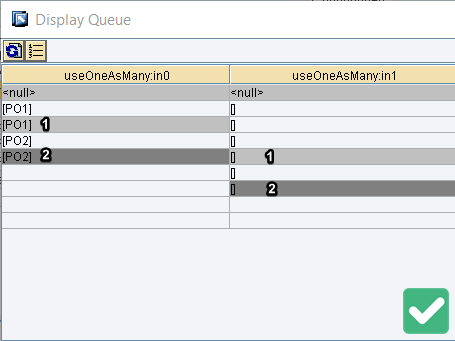# Use of UseOneAsMany Node Function with Examples

## Introduction to Node Function UseOneAsMany.

UseOneAsMany is a node function included in SAP PI/PO Graphical Mapping. UseOneAsMany allows us to repeat a value from source message to multiple segments of the target message. Understanding how this node function operates allows us to avoid creating complex User-Defined Functions (UDFs). For example, if you need to repeat a value of a header segment into multiple repeating target elements, node function UseOneAsMany will be your best option.

The key to learning UseOneAsMany node function is to understand the Input variables and the rules of using them.

#### SAP Versions used in the illustration:

• SAP S4 HANA Fashion 1709
• SAP PO 7.5

## Input and Output of UseOneAsMany Node Function.

UseOneAsMany has 3 inputs or arguments. The output of UseOneAsMany function is derived from these three inputs.

• Input 1: Value from source message which should be repeated at target.
• Input 2: Number of times first argument ‘Input 1’ should be repeated at the target.
• Input 3: Context Change of the target or output.

## Rules of Using the Inputs of UseOneAsMany.

The three Input variables mentioned above should be used in a certain way for the node function to work properly. Here are the rules you need to follow when assigning these three variables from Source message.

• Rule 1: Input 1 should not contain repeating values in the same context.
• Rule 2: Total number of context changes in Input 1 and Input 2 should be equal.
• Rule 3: Number of values in Input 2 should be equal to Input 3.

### Rule 1: Input 1 cannot have repeating values.

Each context of Input 1 should have only 1 value.

Input 1 is the value from the source message that we are trying to repeat in the target, therefore, each context of Input 1 should have only 1 value.

Here are some examples of how to assign the first argument of UseOneAsMany function should be assigned.

In both cases above each context has only 1 value. Values are not repeated.

In this context values are repeated. There are two values in the same context. This is an invalid value assignment for argument 1 of this node function.

### Rule 2: Input 1 and Input 2 Should Have the Same Number of Context Changes.

Number of context changes of Input 1 and Input 2 should be equal.

Here are some examples of correct and incorrect assignment of Input 1 and Input 2 of the node function.Number of context changes in Input 1 and Input 2 are equal. Total of 2 context changes.

### Rule 3: Total Number of Values in Input 2 and Input 3 should be Equal.

Total number of values in Input 2 and Input 3 queues should be equal.

For example, both queues of arguments have 4 values each.

If you follow these rules when assigning the inputs, you will not come across any Queue Exceptions.

## Illustration UseOneAsMany Node Function with Examples.

Let’s look at the use cases of UseOneAsMany node function with a couple of examples.

### Example 1: Repeat Purchase Order Number Using UseOneAsMany.

Let’s assume we have an interface to transfer Purchase Order information. Source message and desired target message are as follows.

Purchase Order (PONumber) should be repeated at target for each line item.

#### UseOneAsMany Mapping:

Input 1 is PONumber in Order context. Argument no 2 is assigned from LineItem and argument no 3 is assigned from ItemNo segment.

Context of the Input 1 <PONumber> is <Orders>.

#### UseOneAsMany Arguments in Detail:

Input 1 does not have any repeating values. Only one purchase order number for each context. First purchase order number PO1 is repeated 3 times and Second purchase order PO2 is repeated once.

Input 3 of the function defines the context changes of the target element. Notice that the total number of values in the Input 2 is equal to the total number of values in Input 3.

### Example 2: Repeat Product ID at Target Message using UseOneAsMany.

Here we have a source message with product header detail. A product can have multiple names. Target message should be created with each product name and corresponding product ID (ProductID).

#### UseOneAsMany Graphical Mapping for ProductID:

Input 1 is ProductID. Input 2 and 3 are both ProductName but third argument uses SplitByValue function to derive the correct context.

#### UseOneAsMany Arguments in Detail:

Product-1 should be repeated twice, while Product-2 has only one product name. Product-3 has three different names, therefore it should be assigned at the target 3 times.

For Input 3 we use SplitByValue node function to define the desired context changes.

Notice that we have adhered to the rules 1, 2 and 3 of UseOneAsMany function. No repeated values in argument 1 which is the Product ID queue.

Argument 2 defines the number of times Product ID should be repeated. Input 2 and 3 contain the same number of context changes in the queue.

Input 2 and 3 have the same number of values.

### Example 3: an Alternative Way of Mapping Product ID.

Let’s take the same source and target messages as in example 2, but use UseOneAsMany node function differently.

#### Alternative Assignment of Node Function Arguments:

We can assign the arguments as below.

I would like to highlight the argument (Input) 3 in this example. Rule 3 is satisfied here as the number of values in Input 2 and input 3 match. We use the SplitByValue after UseOneAsMany to satisfy the context change at the target.

To summarize, we can apply UseOneAsMany node function to repeat values in graphical mapping. There are three input arguments and one output in this function. Assign the input arguments to the function based on the three rules. Hope this article on the different aspects of UseOneasMany node function is helpful to you!

You can also check my previous article on RemoveContext and CollapeContext node functions.

1.•2.•3.•